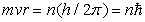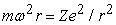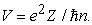﻿ How Many Chemical Elements are there in the Universe? A (not so) <i>Bohring</i> Question

### How Many Chemical Elements are there in the Universe? A (not so) Bohring Question

E. Garrone, C.O. Areán, B. Bonelli

World Journal of Chemical Education

## How Many Chemical Elements are there in the Universe? A (not so) Bohring Question

E. Garrone1, C.O. Areán2, B. Bonelli1,1Department of Applied Science and Technology and INSTM Unit of Torino-Politecnico, Corso Duca degli Abruzzi 24, Politecnico di Torino I-10129, Turin (Italy)

2Departamento de Química, Universidad de las Islas Baleares E-07122, Palma de Mallorca, Spain

### Abstract

This paper replies to two of the most common questions that students usually pose to their teacher during a general chemistry course, i.e. how many chemical elements are in the Periodic Table and how many could be in our Universe. Reply to the former question can be easily found either in the literature or in any updated chemistry book. More interestingly, this communication shows that the latter question may be (simply) answered by making reference to the Bohr’s atomic model that, notwithstanding its well-known limits, allows teachers to demonstrate that (for a hydrogenoid atom) 137 is the highest possible value for Z, as predicted by quantum electrodynamics, a much more complicated theory, usually taught in Physics advanced courses.

• E. Garrone, C.O. Areán, B. Bonelli. How Many Chemical Elements are there in the Universe? A (not so) Bohring Question. World Journal of Chemical Education. Vol. 5, No. 1, 2017, pp 20-22. http://pubs.sciepub.com/wjce/5/1/4
• Garrone, E., C.O. Areán, and B. Bonelli. "How Many Chemical Elements are there in the Universe? A (not so) Bohring Question." World Journal of Chemical Education 5.1 (2017): 20-22.
• Garrone, E. , Areán, C. , & Bonelli, B. (2017). How Many Chemical Elements are there in the Universe? A (not so) Bohring Question. World Journal of Chemical Education, 5(1), 20-22.
• Garrone, E., C.O. Areán, and B. Bonelli. "How Many Chemical Elements are there in the Universe? A (not so) Bohring Question." World Journal of Chemical Education 5, no. 1 (2017): 20-22.

 Import into BibTeX Import into EndNote Import into RefMan Import into RefWorks

1
Prev Next

### 1. Introduction

At the time of grasping the rich wealth of knowledge encoded in the Periodic Table (PT), students of chemistry, frequently, pose the two related questions of how many elements are nowadays in the PT and how many could be in our Universe.

The answer to the first of these questions can easily be found in the literature. To date, there are 118 elements in the PT: the most recent addition (accomplished in 2014) is the element having the atomic number Z = 117 (Ununseptium) , which filled a gap in the island of stability of super heavy elements predicted time ago. [2, 3] Elements 116 and 118 had actually been added earlier, in 2006.  Finally, at the end of 2015 the International Union of Pure and Applied Chemistry (IUPAC) has formally recognized elements 113, 115, 117 and 118.

Of those 118 elements, only 80 are stable, meaning that they all have at least one isotope not undergoing natural decay. There are no known stable isotopes of elements having atomic number greater than that of Pb (Z = 82) (Figure 1), and the same is true for Tc and Pm (atomic number 43 and 61, respective).Download asVeiw figureFigures index
Figure 1. Number of neutrons versus number of protons for (i) stable isotopes belonging to the so-called “band of stability” (green symbols) and (ii) superheavy elements of the “island of stability” (blue symbols), predicted time ago. The vertical red bar points out the maximum predicted value of Z = 137. The dashed black line corresponds to number of neutrons = number of protons

The only known isotope of Bismuth (20083Bi) was thought to be stable until recently; but it turned out to be decaying with a half-life of (1.9±0.2) x 1019 years.  According to current theories of the atomic nucleus, elements yet to be discovered (or produced artificially) are not expected to be stable, if anything because of the well-known instability of atomic nuclei having an ever increasing mass .

The second question above would appear to be more difficult to answer in a simple manner, if anything because the following question arises: besides the instability of atomic nuclei, is there any inherent limit to the number of chemical elements?

### 2. Discussion

The answer to the aforementioned question is given by quantum electrodynamics (QED), a theory that combines quantum mechanics and special relativity, usually taught in Physics advanced courses. The basic idea is easy to grasp: electrons (especially the most internal ones) interacting with a heavy nucleus, showing a correspondingly high nuclear charge, have to move at a high speed to overcome electrostatic attraction. Because there is a limit to any attainable speed (the velocity of light, c), the charge Z of the nucleus cannot be anyone. The result obtained from the rather complex QED theory is, however, simple. The nuclear charge Z cannot exceed 137, i.e., the inverse of the value of the so called fine-structure constant, which is a dimensionless combination of three fundamental constants: e2/ħc, where e is the charge of the electron and ħ is the Plank constant h divided by 2π. The fact that ħc/e2 is very close to an integer number (its approximate value is actually 137.04){1} has in the past stimulated a great deal of speculation.

Nevertheless, for students of Chemistry, who usually are not acquainted with the intricacies of QED, we note herein that the very same result can be arrived at by using the pioneering model proposed by Niels Bohr for the hydrogen atom: actually the first atomic model ever envisaging quantum conditions, and which is present in many textbooks.

In Bohr’s atomic model the electron moves around the nucleus as a planet around the Sun. A circular orbit is assumed (instead of the elliptical orbits later introduced in the Bohr-Sommerfeld model). Because of its 1-D nature, only one quantum number was required in the first model of the hydrogen atom. However, in order to account for multiple discrete energy values for the electron, Bohr assumed that its angular momentum can take values that are an integer multiple of ħ, as shown in equation (1) below,(1)

where m is the mass of the electron, v its speed, r the distance from the centre of the atomic nucleus and n the quantum number.

Let us consider now the case of a nucleus having charge Z. The condition for equality between centrifugal and electrostatic forces acting on an electron orbiting such an atomic nucleus is (in a hydrogenoid system) is given by equation (2) below:(2)

where ω = v/r is the angular velocity and e the charge of the electron.

Criticisms to Bohr’s model are well known and sound, among them:

(i) electrons are not marbles, and they are subjected to the uncertainty principle;

(ii) a charge moving on a bent path radiates energy, which cannot therefore remain constant;

(iii) quantum mechanics expresses the angular momentum in a different way. However, well known are also the merits of Bohr’s model, as it correctly predicts both the radius of the hydrogen atom and the value of the Rydberg constant.

The above criticisms notwithstanding, let us point out that combination of equations (1) and (2) leads to equation (3) below:(3)

Equation (3) shows that, within the Bohr’s atomic model approximation, the speed of the electron is directly proportional to the atomic number and inversely proportional to the (principal) quantum number, so that high velocities would occur for heavy elements and low-lying states. For an atom having atomic number Z*, Eq. (3) shows that the velocity of the electron in its ground state (n = 1) equals the speed of light, c, when Z* = ħc/e2, which is precisely the inverse of the fine structure constant. This means that a hydrogenoid atom having Z* = 137 would have electrons traveling at (approximately) the speed of light, which is forbidden by Relativity. The conclusion is that 137 is the highest possible value for Z, as predicted by QED theory.

### 3. Conclusion

The above simple way of introducing the concept of an inherent limit to the number of chemical elements may prove to be useful when teaching chemistry at a basic level. Moreover, it also proves how far a simple model can sometimes go.

### Notes

1. Note that the value of the fine structure constant (e2⁄ħc) is: 7.297 352 5698(24)x10-3

### References

  Khujagbaatar, J., Yakushev, A., Düllmann, C.E., Ackermann, D., Andersson, L.L., Asai, M., Block, M., Boll, R.A., Brand. H., Cox, D.M., Dasgupta, M., Derkx, X., Di Nitto, A., Eberhardt, K., Even, J., Evers, M., Fahlander, C., Forsberg, U., Gates, J.M., Gharibyan, N., Golubev, P., Gregorich, K.E., Hamilton, J.H., Hartmann, W., Herzberg, R.D., Heßberger, F.P., Hinde, D.J., Hoffmann, J., Hollinger, R., Hübner, A., Jäger, E., Kindler, B., Kratz, J.V., Krier, J., Kurz, N., Laatiaoui, M., Lahiri, S., Lang, R., Lommel, B., Maiti, M., Miernik, K., Minami, S., Mistry, A., Mokry, C., Nitsche, H., Omtvedt, J.P., Pang, G.K., Papadakis, P., Renisch, D., Roberto, J., Rudolph, D., Runke, J., Rykaczewski, K.P., Sarmiento, L.G., Schädel, M., Schausten, B., Semchenkov, A., Shaughnessy, D.A., Steinegger, P., Steiner, J., Tereshatov, E.E., Thörle-Pospiech, P., Tinschert, K., Torres De Heidenreich, T., Trautmann, N., Türler, A., Uusitalo, J., Ward, D.E., Wegrzecki, M., Wiehl, N., Van Cleve, S.M., and Yakusheva, V., “48CIn article  Meyerhof, W.E. Nuclear Structure, Elements of Nuclear Physics. Mc-Graw-Hill, New York, 1967, 11-69.In article  Hofmann, S. “Exploring the island of superheavy elements”, Physics, 3, 31. April 2010.In article  Oganessian, Y.T., Utyonkov, V.K., Lobanov, Y.V., Abdullin, F.S., Polyakov, A.N., Sagaidak, R.N., Shirokovsky, I.V., Tsyganov, Y. S., Voinov, A. A., Gulbekian, G. G., Bogomolov, S. L., Gikal, B. N., Mezentsev, A. N., Iliev, S., Subbotin, V. G., Sukhov, A. M., Subotic, K., Zagrebaev, V. I., Vostokin, G. K. and Itkis, M. G., “Synthesis of the isotopes of elements 118 and 116 in the 249Cf and 245Cm + 48Ca fusion reactions”, Phys. Rev. C, 74, 044602. April 2006.In article  de Marcillac, P., Coron, N., Dambier, G., Leblanc, J., & Moalic, J.P., “Experimental detection of α-particles from the radioactive decay of natural bismuth”, Nature, 422, 876-878. March 2003.In article  Choppin, G., Liljezin, J.O. & Rydberg, J. (2002). Nuclear Mass and Stability, Radiochemistry and Nuclear Chemistry, Butterworth-Heinemann, Woburn, MA, 2002, 41-57.In article